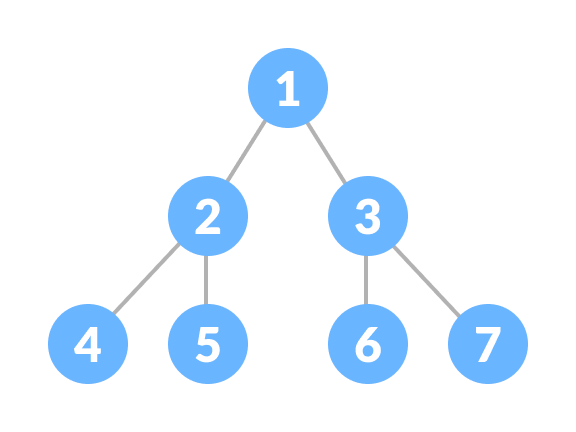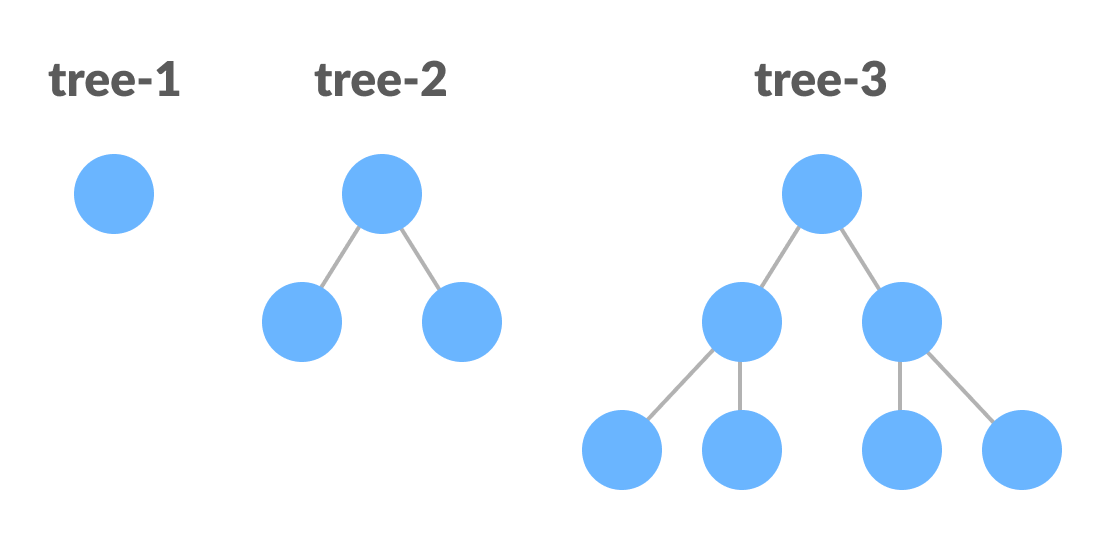Hits: 1

# Perfect Binary Tree

#### In this tutorial, you will learn about the perfect binary tree. Also, you will find working examples for checking a perfect binary tree in C, C++, Java and Python.

A perfect binary tree is a type of binary tree in which every internal node has exactly two child nodes and all the leaf nodes are at the same level.Perfect Binary Tree

All the internal nodes have a degree of 2.

Recursively, a perfect binary tree can be defined as:

1. If a single node has no children, it is a perfect binary tree of height `h = 0`,
2. If a node has `h > 0`, it is a perfect binary tree if both of its subtrees are of height `h - 1` and are non-overlapping.Perfect Binary Tree (Recursive Representation)

## Python Examples

The following code is for checking whether a tree is a perfect binary tree.

``````/* Checking if a binary tree is a perfect binary tree in Python */

class newNode:
def __init__(self, k):
self.key = k
self.right = self.left = None

/* Calculate the depth */
def calculateDepth(node):
d = 0
while (node is not None):
d += 1
node = node.left
return d

/* Check if the tree is perfect binary tree */
def is_perfect(root, d, level=0):

/* Check if the tree is empty */
if (root is None):
return True

/* Check the presence of trees */
if (root.left is None and root.right is None):
return (d == level + 1)

if (root.left is None or root.right is None):
return False

return (is_perfect(root.left, d, level + 1) and
is_perfect(root.right, d, level + 1))

root = None
root = newNode(1)
root.left = newNode(2)
root.right = newNode(3)
root.left.left = newNode(4)
root.left.right = newNode(5)

if (is_perfect(root, calculateDepth(root))):
print("The tree is a perfect binary tree")
else:
print("The tree is not a perfect binary tree")``````

## Perfect Binary Tree Theorems

1. A perfect binary tree of height h has `2h + 1 – 1` node.
2. A perfect binary tree with n nodes has height `log(n + 1) – 1 = Θ(ln(n))`.
3. A perfect binary tree of height h has `2h` leaf nodes.
4. The average depth of a node in a perfect binary tree is `Θ(ln(n))`.

# Special 95% discount

## 2000+ Applied Machine Learning & Data Science Recipes

### Portfolio Projects for Aspiring Data Scientists: Tabular Text & Image Data Analytics as well as Time Series Forecasting in Python & R## Two Machine Learning Fields

There are two sides to machine learning:

• Practical Machine Learning:This is about querying databases, cleaning data, writing scripts to transform data and gluing algorithm and libraries together and writing custom code to squeeze reliable answers from data to satisfy difficult and ill defined questions. It’s the mess of reality.
• Theoretical Machine Learning: This is about math and abstraction and idealized scenarios and limits and beauty and informing what is possible. It is a whole lot neater and cleaner and removed from the mess of reality.

Data Science Resources: Data Science Recipes and Applied Machine Learning Recipes

Introduction to Applied Machine Learning & Data Science for Beginners, Business Analysts, Students, Researchers and Freelancers with Python & R Codes @ Western Australian Center for Applied Machine Learning & Data Science (WACAMLDS) !!!

Latest end-to-end Learn by Coding Recipes in Project-Based Learning:

Applied Statistics with R for Beginners and Business Professionals

Data Science and Machine Learning Projects in Python: Tabular Data Analytics

Data Science and Machine Learning Projects in R: Tabular Data Analytics

Python Machine Learning & Data Science Recipes: Learn by Coding

R Machine Learning & Data Science Recipes: Learn by Coding

Comparing Different Machine Learning Algorithms in Python for Classification (FREE)

`Disclaimer: The information and code presented within this recipe/tutorial is only for educational and coaching purposes for beginners and developers. Anyone can practice and apply the recipe/tutorial presented here, but the reader is taking full responsibility for his/her actions. The author (content curator) of this recipe (code / program) has made every effort to ensure the accuracy of the information was correct at time of publication. The author (content curator) does not assume and hereby disclaims any liability to any party for any loss, damage, or disruption caused by errors or omissions, whether such errors or omissions result from accident, negligence, or any other cause. The information presented here could also be found in public knowledge domains.  `